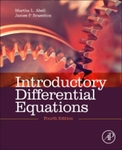## Mathematical Sciences Faculty Bookshelf#### Title

Introductory Differential Equations

#### Description

Georgia Southern faculty members Martha L. Abell and James P. Braselton co-authored Introductory Differential Equations.

Book Summary:

This text is for courses that are typically called (Introductory) Differential Equations, (Introductory) Partial Differential Equations, Applied Mathematics, and Fourier Series. Differential Equations is a text that follows a traditional approach and is appropriate for a first course in ordinary differential equations (including Laplace transforms) and a second course in Fourier series and boundary value problems.

Some schools might prefer to move the Laplace transform material to the second course, which is why we have placed the chapter on Laplace transforms in its location in the text. Ancillaries like Differential Equations with Mathematica and/or Differential Equations with Maple would be recommended and/or required ancillaries.

Because many students need a lot of pencil-and-paper practice to master the essential concepts, the exercise sets are particularly comprehensive with a wide range of exercises ranging from straightforward to challenging. Many different majors will require differential equations and applied mathematics, so there should be a lot of interest in an intro-level text like this. The accessible writing style will be good for non-math students, as well as for undergrad classes.

2014

#### Publication Title

Introductory Differential Equations

Waltham, MA

#### ISBN for this edition (13-digit)

9780124172197COinS